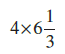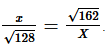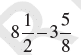Welcome to your Level 03 Practice VM Sr

Name
1.
Divide 7714 by 67 using straight division method

2.
Find 12% of 150 by using ratio proportion equation

3.
Rohit solved 2/7 part of an exercise while Sanskruti solved 4/5 of it. Who solved lesser part?

4.
Find the value of :5.
Find the ratio of 3 km to 300 m.

6.
Out of 25 children in a class, 15 are girls. What is the percentage of girls?

7.
Find the square root of 298116 by general vedic method.

8.
Which of the following number is divisible by 11

9.
Is it necesary that a number divisible by 14 and 7 , will also be divisible by 21?

10.
Find the squre root of 7744 by estimation method.

11.
Product of two co prime number is 913. Their LCM will be_________

12.
What should come in place of both x in the equation:13.
LCM of two numbers is six times their HCF. If HCF is 12 and one number is 36 find the other number

14.
Find the least number of 6 digits which is a perfect square.

15.
After decreasing 24% in the cost price of an article,its costs Rs.912. Find the actual cost of an article?

16.
Find the cube root of 250047.

17.
Divide 136878 by 1122?

18.
Find the cube root of 571787 by vedic method.

19.
Convert 0.75 to per cents:

20.
Find the HCF and LCM of 28 and 72 by vertical and cross wise method.

21.
Is 53240 a perfect cube? If not, then by which smallest natural number should 53240 be divided so that the quotient is a perfect cube?

22.
In a class each of the students contributed as many paise as there are number of students. If the total collection was ₹ 81, what is the number of students in the class?

23.
Find the value of :24.
₹6400 are divided among three workers in the ratio 3/5:2:5/3. The share of the Second worker is:

25.
Three -fifth of the square of a number is 126.15. What is the number: